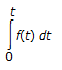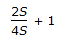# Chemical Engineering - Process Control and Instrumentation

### Exercise :: Process Control and Instrumentation - Section 7

36.

For the time domain function f(t) = t, the Laplace transform ofis given by

 A. 1/2 S3 B. 2/S3 C. 1/S3 D. 2/S2

Explanation:

No answer description available for this question. Let us discuss.

37.

Temperature of __________ can not be measured by an optical or radiation pyrometer.

 A. hot blast (air) from stoves B. molten slag flowing out of blast furnace C. combustion space in boilers D. rotary limestone calcination kiln

Explanation:

No answer description available for this question. Let us discuss.

38.

Gain margin is equal to the

 A. amplitude ratio B. reciprocal of amplitude ratio C. gain in P controller D. gain in P-I controller

Explanation:

No answer description available for this question. Let us discuss.

39.

A system with transfer functionis of __________ order.

 A. zero B. 1st C. 2nd D. 3rd

Explanation:

No answer description available for this question. Let us discuss.

40.

In Bode plot, Φ vs ω is plotted on a/an __________ graph paper.

 A. log-log B. ordinary C. semi-log D. triangular